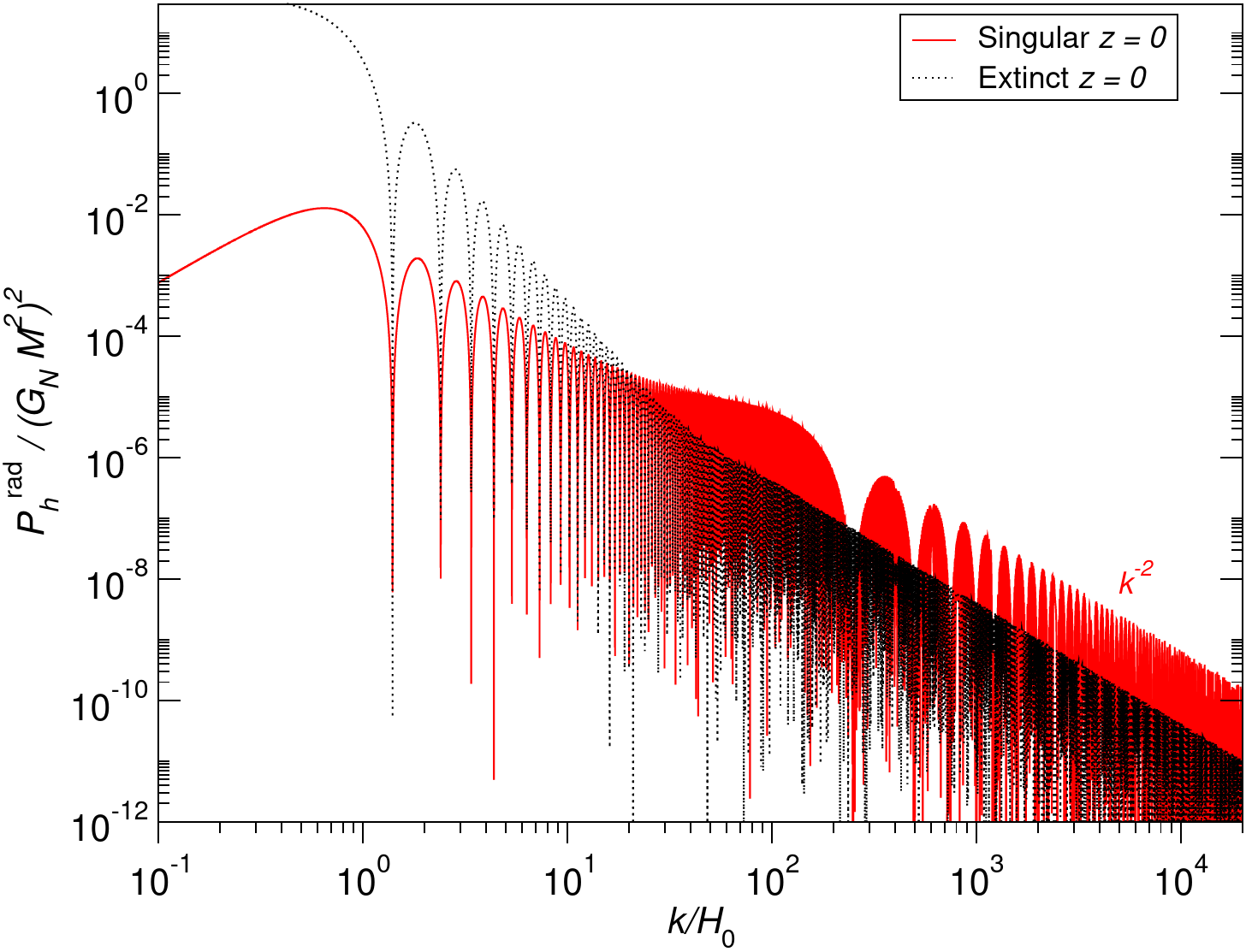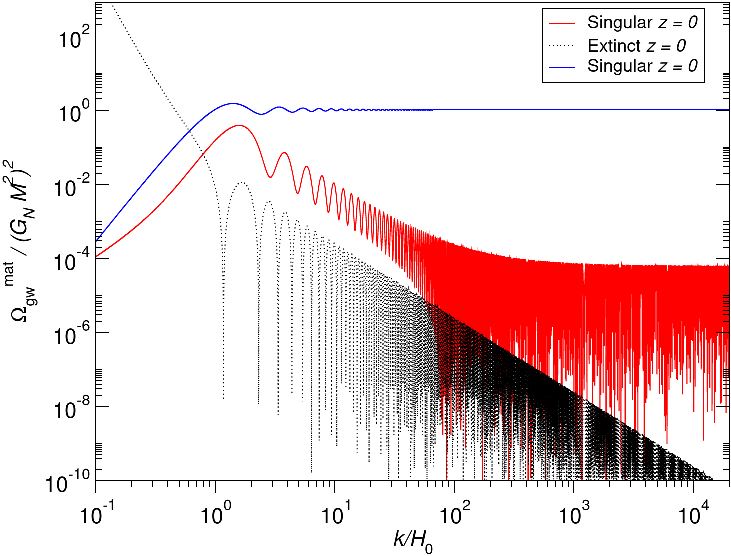# Interferences in the Stochastic Gravitational Wave Background

Some cosmological sources of gravitational waves, such as cosmic strings, are everywhere present and incessantly emitting. Their past and present contribution can mix together to create some fine structure in the stochastic gravitational wave background today.

Disrael and Christophe have calculated in Ref.  the all-length scales unequal-time strain power spectrum $$\mathcal{P}_h$$ and the energy density parameter $$\Omega_{\mathrm{GW}}$$ of gravitational waves generated by various scaling sources. For extinct and smooth enough sources, they find that both quantities have an oscillatory fine structure, represented as black dotted curve in the following figures.On top of that, singular and never extinct sources, such as cosmic strings, can create interferences and their spectra exhibit a different fine structure. They are represented as red curves in the figures. For instance, long cosmic strings in the matter era are expected to have a rescaled strain spectrum $$k^2 \mathcal{P}_h^{\mathrm{mat}}$$ oscillating around a scale invariant plateau (blue curve above), which is quite different from the one associated with extinct and smooth sources. It also deviates significantly from $$\Omega_{\mathrm{GW}}^{\mathrm{mat}}$$.

Will the fine structure of the stochastic gravitational wave background be measured?

### References

•  Cunha D C N da and Ringeval C 2021 Interferences in the Stochastic Gravitational Wave Background JCAP 2108 005
Abstract: arXiv:2104.14231 10.1088/1475-7516/2021/08/005